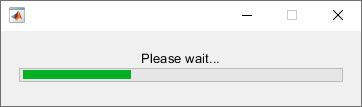Documentation

### This is machine translation

Mouseover text to see original. Click the button below to return to the English version of the page.

Note: This page has been translated by MathWorks. Click here to see
To view all translated materials including this page, select Country from the country navigator on the bottom of this page.

# send

Send data from worker to client using a data queue

## Syntax

``send(queue, data)``
``send(pollablequeue, data)``

## Description

example

````send(queue, data)` sends a message or data with the value `data` to the `parallel.pool.DataQueue` specified by `queue`. Call `afterEach` to pass each of the pending messages to the function specified by `afterEach`. ```

example

````send(pollablequeue, data)` sends a message or data with the value `data` to the `parallel.pool.PollableDataQueue` specified by `pollablequeue`. Retrieve the result using `poll``(pollablequeue)`, and return `data` as the answer.Use the `send` and `poll` functions together using a pollable data queue to transfer and retrieve messages or data from different workers.```

## Examples

collapse all

Construct a `DataQueue`, and call `afterEach`.

```q = parallel.pool.DataQueue; afterEach(q, @disp); ```
Start a `parfor`-loop, and send a message. The pending message is passed to the `afterEach` function, in this example `@disp`.

```parfor i = 1:3 send(q, i); end;```
``` 1 2 3```

For more details on listening for data using a `DataQueue`, see `afterEach`.

Construct a `PollableDataQueue`.

```p = parallel.pool.PollableDataQueue; ```
Start a `parfor`-loop, and send a message, such as data with the value 1.
```parfor i = 1 send(p, i); end ```
Poll for the result.

`poll(p)`
``` 1 ```

For more details on retrieving data using a `PollableDataQueue`, see `poll`.

This example shows a function that creates a `parfor` wait bar. Create a `DataQueue`, and use `afterEach` to specify the function to execute each time the queue receives data. This example calls a subfunction that updates the wait bar.

Create a `parfor`-loop to carry out a computationally demanding task in MATLAB®. Use `send` to send some dummy data on each iteration of the `parfor`-loop. When the queue receives the data, `afterEach` calls `nUpdateWaitbar` in the client MATLAB, and you can observe the wait bar progress.

```function a = parforWaitbar D = parallel.pool.DataQueue; h = waitbar(0, 'Please wait ...'); afterEach(D, @nUpdateWaitbar); N = 200; p = 1; parfor i = 1:N a(i) = max(abs(eig(rand(400)))); send(D, i); end function nUpdateWaitbar(~) waitbar(p/N, h); p = p + 1; end end ```## Input Arguments

collapse all

Data queue, specified as a `parallel.pool.DataQueue` object.

Example: `q = parallel.pool.DataQueue;`

Message or data from workers to a data queue, specified as any data type that can be serialized.

Example: `send(queue, data);`

Pollable data queue, specified as a `parallel.pool.PollableDataQueue` object.

Example: `p = parallel.pool.PollableDataQueue;`

Watch now